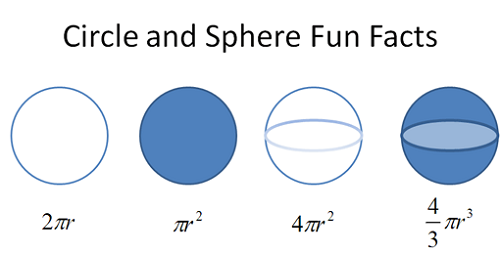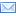#Difference Between Similar Terms and Objects

# Difference Between Degrees and Radians

### Degrees vs Radians

Geometry involves the study of shapes, sizes, positions, and properties of space. It is also concerned with lengths, areas, volumes, and other measurements of space, lines, points, and planes. It studies dimensions and symmetry in patterns such as those found in solids, polygons, and the circle. A circle has a radius, a diameter, and a circumference. It also contains angles.Angles are geometric figures which are formed by two rays connected at a common point or vertex. When two lines meet each other at a certain point, an angle is formed, and its measurement varies according to the rotation of the lines or rays about the vertex. In measuring angles, there are several units used, but the most common are degrees and radians. They are also the units used in measuring circles and spheres to determine their area, volume, perimeter, and circumference.

Degrees are units of measurements which are used to determine the size of an angle and express its direction. When an angle goes all the way around in a circle, it is equivalent to 360 degrees. The 360 degrees of a circle is the basis for the calculation of angle measurements. When measuring the angle of a part of a circle, it starts at the center and extends to its perimeter. The angles of a triangle, on the other hand, are equal to 180 degrees.

Degree calculations often involve larger numbers, and they can be a little complicated. Degrees are taught early in school when students are taught simple mathematics, usually in elementary school then continuing in high school and college.

The other common unit of measuring angles, the radian, on the other hand, is taught in the later part of high school and then in college. It is used in higher or advanced mathematics such as trigonometry and calculus. Calculations using radians involve smaller numbers, and they are an easier and simpler way to calculate angles. The radius of a circle is one-half of the distance across its center which makes an angle equal to one radian. A radian is equal to 180 degrees because a whole circle is 360 degrees and is equal to two pi radians.

A radian is not as widely used in the measurement of circles and angles as a degree because it involves the knowledge of higher mathematics and includes tangents, sine, and cosines which are taught in college.

### Summary:

1.A degree is a unit of measurement which is used to measure circles, spheres, and angles while a radian is also a unit of measurement which is used to measure angles.
2.Degrees are taught early in school beginning in elementary school then high school and up to college while radians are taught later usually in senior high school and college.
3.Of the two, degrees are used more commonly as they use simple mathematics while radians use higher or advanced mathematics.
4.A circle has 360 degrees which is its full area while its radian is only half of it which is 180 degrees or one pi radian

### Search DifferenceBetween.net :

Custom Search

Help us improve. Rate this post!(2 votes, average: 5.00 out of 5)Loading...Email This Post : If you like this article or our site. Please spread the word. Share it with your friends/family.

### 5 Comments

1. Another very important difference between radians & degrees is needed when you are considering rates of change (calculus) When you differentiate sine x, you get cosine x. This only works in radians because of the need for small increments. As the change in x (delta x) tends to zero, the value of sine x is equal to x but this is only true when the angle is measured in radians.
I suppose this application of mathematics is your higher mathematics.

2. Just a important note to all who are reading, one half of a circle is equal to pi radians or 3.14… radians, if you will. One radian is equal to 57 degrees, more or less.

One half of a circle is NOT equal to one radians.

• 180 / pi = 1 Radian = 57.2957~ °.
Degrees are a measure of angle, whereas a Radian is a measure of length. A Radian is equal to the length of the Radius of a circle, but curved to fit exactly on top of a portion of the circumference of that circle. It happens that to close (or cover the circumference of) the circle with Radians requires 6 Radians + (.2832 x’s the Radius).

A circle with a Diameter of 30 and a Radius of 15:
30 x pi = a circumference of 94.2477~
(15 x 6) + 0.2832 x 15) = 94.248 = 6 x’s the Radius + 0.2832 of the Radius

3. Jez D is pointing out that the phrase “A radian is equal to 180 degrees” is incorrect. Correct is “Pi radians are equal to 180 degrees”.

4. All the comments are vulgarly incorrect and make me gag.

Please note: comment moderation is enabled and may delay your comment. There is no need to resubmit your comment.

Articles on DifferenceBetween.net are general information, and are not intended to substitute for professional advice. The information is "AS IS", "WITH ALL FAULTS". User assumes all risk of use, damage, or injury. You agree that we have no liability for any damages.

See more about : ,